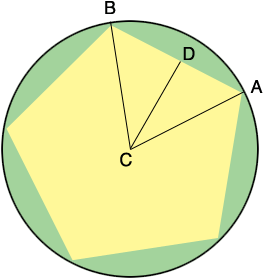SEARCH HOMEMath Central Quandaries & QueriesQuestion from Jamie, a student: A regular pentagon is inscribed in a circle of radius 4.5 cm. Determine its perimeter and area to one decimal place! Thank YOU ! :)Hi Jamie,The measure of angle BCA is 360/5 = 72o. D is the midpoint of AB so angle ADC is a right angle. What is the measure of angle DCA? A little trigonometry tells you the rest.

PennyMath Central is supported by the University of Regina and The Pacific Institute for the Mathematical Sciences.